## Example Questions

← Previous 1 3

### Example Question #33 : Triangles

Two interior angles in an obtuse triangle measure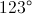and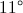. What is the measurement of the third angle.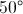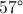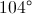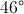Explanation:

Interior angles of a triangle always add up to 180 degrees.

### Example Question #2 : How To Find The Measure Of An Angle

In a given triangle, the angles are in a ratio of 1:3:5.  What size is the middle angle?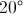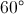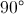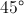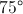Explanation:

Since the sum of the angles of a triangle is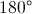, and given that the angles are in a ratio of 1:3:5, let the measure of the smallest angle be, then the following expression could be written: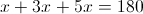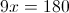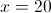If the smallest angle is 20 degrees, then given that the middle angle is in ratio of 1:3, the middle angle would be 3 times as large, or 60 degrees.

### Example Question #166 : Plane Geometry

In the triangle below, AB=BC (figure is not to scale) .  If angle A is 41°, what is the measure of angle B?

A (Angle A = 41°)B                           C

82

98

41

90

98

Explanation:

If angle A is 41°, then angle C must also be 41°, since AB=BC.  So, the sum of these 2 angles is:

41° + 41° = 82°

Since the sum of the angles in a triangle is 180°, you can find out the measure of the remaining angle by subtracting 82 from 180:

180° - 82° = 98°

### Example Question #171 : Act Math

Points A, B, C, D are collinear. The measure of ∠ DCE is 130° and of ∠ AEC is 80°. Find the measure of ∠ EAD.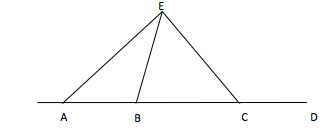50°

70°

60°

80°

50°

Explanation:

To solve this question, you need to remember that the sum of the angles in a triangle is 180°. You also need to remember supplementary angles. If you know what ∠ DCE is, you also know what ∠ ECA is. Hence you know two angles of the triangle, 180°-80°-50°= 50°.

### Example Question #1 : How To Find An Angle In An Acute / Obtuse Triangle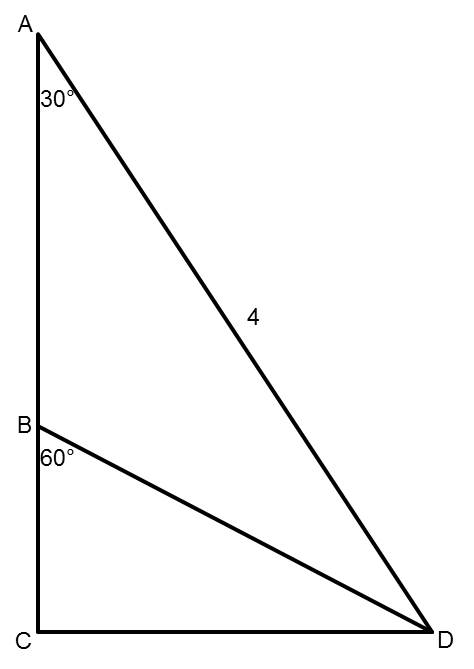Points A, B, and C are collinear (they lie along the same line). The measure of angle CAD is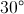. The measure of angle CBD is. The length of segment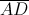is 4.

Find the measure of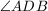.Explanation:

The measure ofis. Since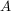,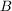, and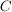are collinear, and the measure of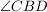is, we know that the measure of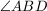is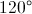.

Because the measures of the three angles in a triangle must add up to, and two of the angles in triangle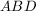areand, the third angle,, is.

### Example Question #172 : Plane Geometry

Observe the following image and answer the question below:Are triangles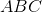and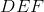congruent?

Not enough information to decide.

Maybe

No

Yes

Yes

Explanation:

Two triangles are only congruent if all of their sides are the same length, and all of the corresponding angles are of the same degree. Luckily, we only need three of these six numbers to completely determine the others, as long as we have at least one angle and one side, and any other combination of the other numbers.

In this case, we have two adjacent angles and one side, directly across from one of our angles in both triangles. This can be called the AAS case. We can see from our picture that all of our angles match, and the two sides match as well. They're all in the same position relative to each other on the triangle, so that is enough information to say that the two triangles are congruent.

### Example Question #31 : Triangles

Two similiar triangles have a ratio of perimeters of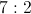.

If the smaller triangle has sides of 3, 7, and 5, what is the perimeter of the larger triangle.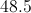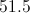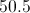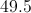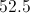Explanation:

Adding the sides gives a perimeter of 15 for the smaller triangle. Multipying by the given ratio of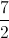, yields 52.5.

### Example Question #174 : Plane Geometry

Two similiar triangles exist where the ratio of perimeters is 4:5 for the smaller to the larger triangle. If the larger triangle has sides of 6, 7, and 12 inches, what is the perimeter, in inches, of the smaller triangle?

18

25

23

20

20

Explanation:

The larger triangle has a perimeter of 25 inches. Therefore, using a 4:5 ratio, the smaller triangle's perimeter will be 20 inches.

### Example Question #1 : How To Find The Perimeter Of An Acute / Obtuse Triangle

Two similar triangles' perimeters are in a ratio of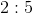. If the lengths of the larger triangle's sides are,, and, what is the perimeter of the smaller triangle?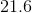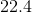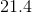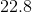Explanation:

1. Find the perimeter of the larger triangle: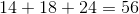2. Use the given ratio to find the perimeter of the smaller triangle: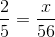Cross multiply and solve: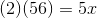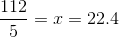### Example Question #176 : Plane Geometry

There are two similar triangles. Their perimeters are in a ratio of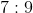. If the perimeter of the smaller triangle is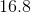, what is the perimeter of the larger triangle?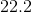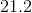Explanation:

Use proportions to solve for the perimeter of the larger triangle: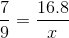Cross multiply and solve: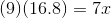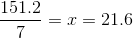← Previous 1 3